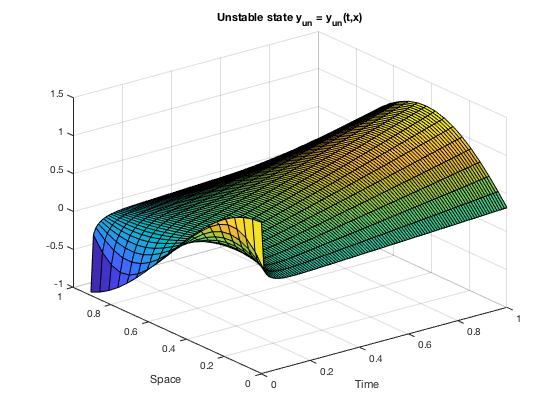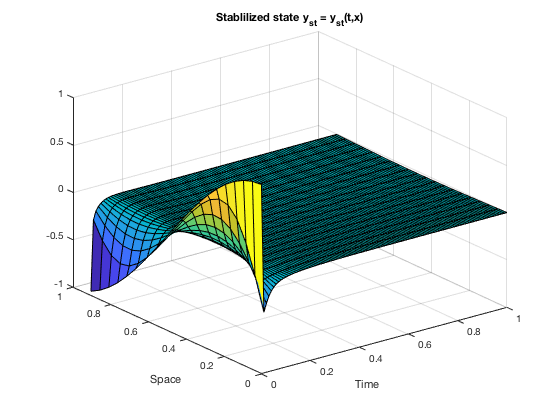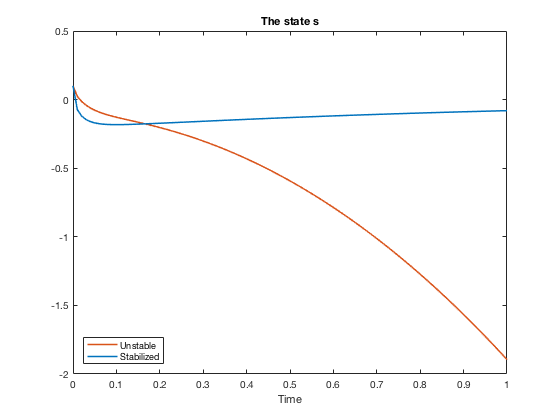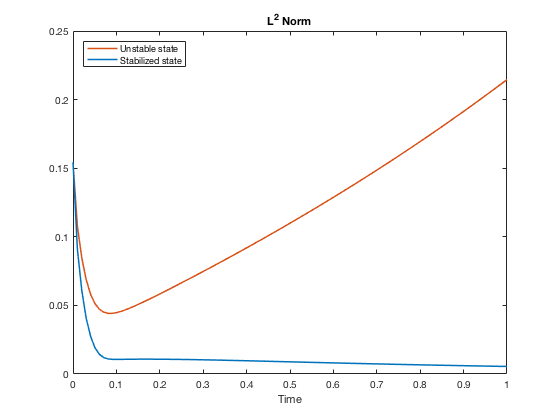# LQR optimal control design for a coupled PDE-ODE system.

Author: - 28 October 2018

In this tutorial, we will demonstrate how to design a LQR controller in order to stabilize the following linear coupled (hybrid) PDE-ODE system:

Similar systems may appear in the modelling of a variety physical phenomena, such as the melting of ice in a body of water  or fluid-structure interaction . This particular system may be seen as a simplification of the well known one-phase Stefan problem. The stabilization and control of the former has been investigated in recent works by Krstic et al. (see  for example). The relationship between the considered system and the Stefan problem, as well as the control of the latter will be considered in a future tutorial.

When no forces are applied, a feature of the governing parabolic PDE is its possibility of generating unstable dynamics as $t \rightarrow \infty$. This phenomenon is due to the fact that the sign of the eigenvalues of the governing elliptic differential operator depends on the magnitude of the constant $\beta$. More precisely, as the differential operator has eigenvalues of the form $\mu_k = \beta - \lambda_k$, where $\lambda_k$ are the eigenvalues of the Dirichlet laplacian, taking $\beta > \lambda_1$ (the biggest among the $\lambda_k$) would yield such a result. We will thence implement a LQR optimal control strategy in order to stabilize the system.

We first need to reduce the PDE-ODE system to a finite dimensional system of the form

where $A$ and $B$ are matrices, and

is the finite dimensional state vector.

We fix the parameters as follows: $T=1$, $\alpha=1$ and $\beta = 10$:

clc; clear;
T = 1;
nT = 100; nX = 20;
dt = T/nT; dx = 1/(nX+1);
x = linspace(0,1,nX+2);
Dir0 = 1; Dir1 = 0;
alpha = 1; beta = 10;


We begin the code by discretizing in space the parabolic PDE; this is done by finite differences:

Id = eye(nX);
Lapl = -2*Id + diag(ones(nX-1,1),-1) + diag(ones(nX-1,1),1);
A0 = alpha*1/dx^2*Lapl + beta*Id;


In fact, the finite difference discretization of the Dirichlet laplacian may also be done using the MATLAB routine delsq. We proceed similarly for the term $y_x(1,t)$ in the ODE, and assemble the complete matrix $A$:

A = zeros(nX+1,nX+1); A(2:nX+1,2:nX+1) = A0; A(1,2)=-1/dx;


The control matrix B is constructed as follows:

b = zeros(nX,1); b(1,1) = alpha*Dir0/dx^2; b(nX,1) = alpha*Dir1/dx^2;
B = zeros(nX+1,1);
B(1,1) = Dir0/dx;
B(2:nX+1,1) = b;


We may now proceed with the optimal control strategy. For a continuous time linear system generated by the matrices $(A,B)$ and an infinite time horizon cost functional defined as

the feedback control law that minimizes the value of the cost is $u = -Kz$, where $K = R^{-1}B*P$ and $P$ is found by solving the continuous time algebraic Ricatti equation

We check whether the constructed matrices fit in the framework of LQR. Namely, we first verify that the couple $(A, BB*)$ is stabilizable. This is done by checking if $\text{rank}[\lambda I - A, B] = d$ for any $\lambda \in \sigma(A)\cap \mathbb{R}_+$, where $d$ is the dimension of the state space.

eigs = eig(A);
for iter=1:nX+1
if real(eigs(iter))>=0
M = zeros(nX+1,2*(nX+1));
M(:,1:nX+1) = eigs(iter)*eye(nX+1)-A; M(:,nX+2:2*(nX+1)) = B*(B');
r_ = rank(M);
stabilizable = (r_ == nX+1);
end
end


Secondly, we check that the eigenvalues of the associated Hamiltonian matrix do not lie on the imaginary axis.

R = 1; Q = eye(nX+1);
H = blkdiag(A, -A');
H(nX+2: 2*(nX+1), 1:nX+1) = -Q; H(1:nX+1, nX+2: 2*(nX+1)) = -B*(B');
eigH = real(eig(H));
nb_im_eig = sum(eigH == 0);


Finally, we check if the pair $(Q, A)$ is detectable; the variable not_det should equal $0$ after the loop.

not_det = 0;
for iter_=1:nX+1
if real(eigs(iter_))>=0
M_ = zeros(2*(nX+1), nX+1);
M_(1:nX+1, :) = Q; M_(nX+2:(nX+1)*2, :) = eigs(iter_)*eye(nX+1)-A;
r_ = rank(M_);
if (r_ ~= nX+1)
not_det = not_det + 1;
end
end
end


We are now in a position to construct the LQR feedback control by solving the algebraic Ricatti equation. This is done by using the MATLAB Control Toolbox routine care:

[ricsol, cleig, K, report] = care(A, B, Q, R);
f_ctr = @(z) -K*z;


We now compare the uncontrolled (thence unstable) dynamics with the dynamics stabilized by the LQR feedback control. As initial datum for both systems, we pick $u_0(x) = \cos(\pi*x)$ and $s_0 = 0.1$.

x_int = x(1:nX);
y0 = cos(x_int*pi)';
z0 = zeros(nX+1,1); z0(1,1) = 0.1; z0(2:nX+1,1) = y0;


The free system is solved with the Dirichlet boundary condition $u(0,t) = 0.15$:

f1 = @(t,v) A*v + B*0.15;
[t1, z_free] = ode45(f1, linspace(0,T,nT), z0);
[X1,Y1] = meshgrid(t1,x_int);
surf(X1, Y1, z_free(:,2:nX+1)');
xlabel('Time');
ylabel('Space');
title('Unstable state y_{un} = y_{un}(t,x)')Now we solve and visualize the controlled problem:

f2 = @(t,z) A*z + B*f_ctr(z);
[t2, z_ctr] = ode45(f2, linspace(0,T,nT), z0);
figure;
[X2,Y2] = meshgrid(t2,x_int);
surf(X2, Y2, z_ctr(:,2:nX+1)');
xlabel('Time');
ylabel('Space');
title('Stablilized state y_{st} = y_{st}(t,x)')We also plot the corresponding solutions to the ODE:

figure;
plot(t1, z_free(:,1), 'Color', [0.8500, 0.3250, 0.0980], 'LineWidth', 1.5);
hold on;
plot(t2, z_ctr(:,1), 'Color', [0, 0.4470, 0.7410], 'LineWidth', 1.5);
legend('Unstable', 'Stabilized', 'Location', 'SouthWest');
xlabel('Time')
title('The state s')As a final test of our results, we compare the $L^2(0,1)$-norms of both the controlled and uncontrolled state with respect to time.

norm_free = 1/nX*sum(z_free.^2,2).^0.5;
norm_ctr = 1/nX*sum(z_ctr.^2,2).^0.5;
figure;
plot(t1, norm_free, 'Color', [0.8500, 0.3250, 0.0980], 'LineWidth', 1.5);
hold on;
plot(t2, norm_ctr, 'Color', [0, 0.4470, 0.7410], 'LineWidth', 1.5);
legend('Unstable state', 'Stabilized state', 'Location', 'NorthWest');
xlabel('Time');
title('L^2 Norm');Koga, Shumon and Diagne, Mamadou and Krstic, Miroslav. Output feedback control of the one-phase Stefan problem. 2016 IEEE 55th Conference on Decision and Control (CDC) (2016).

 Vazquez, Juan Luis and Zuazua, Enrique. Large time behavior for a simplified 1D model of fluid-solid interaction. Comm. Partial Differential Equations 28 (2003), no. 9-10, 1705-1738.